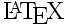# tex2imgsrc: (La)TeX expression to <img src...>

Script here: tex2imgsrc

## What's this? / $B$J$K$9$k$b$N$>(B

Convertcoded sentences to image file and output <img src="..."> notation to standard output. It's very handy to write formulas in HTML especially with SSI. This script is for the people who are disappointed to MathML because of its terrible complexity.

$B$b$7(BHTML$B=q$-$K(Byahtml $B$r;H$C$F$$kJiJQ?t(B yahtml-lint-program BK(B "./tex2imgsrc -s" Br%;%C%H7F*1P(B [prefix] t j BG0lH/JQ49G-^9!#(B Id: yahtml.el,v efc3819f749b 2010-09-12 10:59 +0900 yuuji  B0J9_N(ByahtmlBJi(BHTMLB%=!<%9N@hF,IU6aK(B <!-- #lint ./tex2imgsrc -s -->  BHF~lF*1P<+F0E*KMxMQ5l^9(B(-sBNItJ,,(B&#45;sBGb(BOK)B!#(B BJs@!"(BWebBK?t<0=q/N,6lDK8cJ/JC?h!#(B ## Without SSI / SSIB,I&7Fb;H(J$$$1$I(B...

You can use SSI notation, '<!--#exec cmd="..."-->' even if your server does not support SSI. Expand all SSI lines in shtml beforehand by tex2imgsrc -S'.

tex2imgsrc -S src.shtml > index.html


SSI$B$,;H$($J$$%5!<%PGb!"(BSSIBMQK=q$$$?5-K!$rE83+$9$k(B tex2imgsrc -S' $B$r;H$($P2hA|:n@.$H(Bimg$B%?%0$X$NE83+$r;vA0$K9T$J$($^$9!#(B $B>e5-$N%3%^%s%I%i%$%sNc$N$h$&$K$7$F$*$/$3$H$G!"(Bsrc.shtml $B$r(BSSI$BE83+$7$?7k2L$,(B index.html $B$K=q$-9~$^$l!"F1;~$K2hA|@8@.$b(B $B9T$J$o$l$^$9!#(B ## Requirements / $BI,MW$J%D!<%k(B This script requires the following utilities. • POSIX shell (sh) • LaTeX2e (\usepackage{color} ready) • dvips -E (for generate EPS) • Image converter either of NetPBM or ImageMagick • Ruby (required for tex2imgsrc -s/-S) • sed, grep, head ## Inside / $B;EAH(B

If you can't make tex2imgsrc work well, try these steps.

1. Check color capability of LaTeX2e.

color.tex

\documentclass{article}
\pagestyle{empty}
\usepackage{color}
\begin{document}
\textcolor{red}{Tomato!}
\end{document}

2. See the color.
xdvi color.dvi

3. Convert it to EPS.
dvips -E -o color.eps color.dvi
gs color.eps  (for confirmation)

4. Convert EPS to png/jpg.
convert -density 200 color.eps color.png
(or)
pstopnm -stdout -dpi=200 -portrait color.eps | pnmtopng > color.png


Generally, troubles occur at conversion processes. Please check configuration of dvips or ImageMagick.

## Options / $B%*%W%7%g%s(B -e echo only(no exec) $B%W%m%;%95/F0$;$:I=<($N$_(B
-s file(s)      exec all SSIs in HTML files     shtml$B$rFI$s$G0l3g2hA|@8@.(B
-S SHTML >HTML  Expand SSI lines in SHTML       shtml$B$r(Bhtml$B$KJQ49(B
-p PT           pt size of document             LaTeX$B%I%-%e%a%s%H$N(Bpt$B;XDj(B -d DPI set eps DPI (200) EPS$B$N(Bdpi$BCM(B 200$B$,%G%U%)%k%H(B
-t TEXCMD       set latex command               $B;HMQ$9$k%?%$%W%;%C%?(B
-F COLOR        set textcolor                   $BJ8;z?'(B -B COLOR set background color $BGX7J?'(B
-c CLS          set documentclass(jarticle)
-C CLASS        set css-class name (teximg)     img$BMWAG$KIU2C$9$k%/%i%9L>(B


Colors can be specified by basic color, named color, rgb/cmyk specs. The basic colors are black, blue, cyan, green, magenta, red, white and yellow. The named colors are such as Apricot, Aquamarine, Bittersweet and so on defined in colordvi.tex, which may be seen as below.

less kpsewhich colordvi.tex


The rgb/cmyk values are specified as comma-separated triple/quadruple real numbers between 0 and 1. Thus, these color specs for -F (or -B) option below are all acceptable.

-F white
-F VioletRed
-F 0.2,0.4,0.5
-F 0.9,0.8,0.5,0.2


Options are passed also via environment variable. The textcolor, for example, is passed by $FG. So these two lines are equivalent. FG=BlueGreen tex2imgsrc '$y=\sin(\theta)$' yst.png tex2imgsrc -FBlueGreen '$y=\sin(\theta)$' yst.png  This can be convenient when you specify same options to all tex2imgsrc commands in a HTML documents. If you decide to specify -F Brown -d 180' for all images, make a wrapper to tex2imgsrc like this: #!/bin/sh exec env - PATH=$PATH FG=Brown DPI=180 ./tex2imgsrc "$@"  Then, rewrite all tex2imgsrc' in SSI-exec lines to the name of wrapper above. More environment variables are available. Please take a look at the beginning part of script. ## Consideration / SSI$B$C$FJ?5$(B? Perhaps you may see the notes on SSI security issue, do not worry if you write .shtml files manually. SSI$B$r0G1@$K4m81$H=q$$F"k%Z!<%8r8++1k+b7l^;s,!"(B B3lO4IM}l9gNLdBjG"CF!"(B SSIBr4^%U%!%%krK3Nh&J%9%/%j%W%Hr(BCGIBH7F(B B@_CV9kH%/%m%9%5%%H%9%/%j%W%F%#%s%0N4m81K;/5lkNG(B BhC]I4m81G9!#(B Bb7(BSSIBr5v2D7F/lk%5!<%P,J/F*:$$j$J$i!"%7%'%k$N;H$($k(B gentei.org $B$r$I$&$>(B:-) ## Want to use, but doesn't work / $B;H$$?$$$1$IF0$+$M(B

I'll try to fix your problem. Send me a report with detail.

$BD>$7$^$9(B($B$?$V$s(B)$B!#$G$-$k$@$1>u67$r>\$7\$/=q$$FO"Mm2<5$$!#(B

yuuji@Fingerprint16 = FF F9 FF CC E0 FE 5C F7 19 97 28 24 EC 5D 39 BA
HIROSE Yuuji - ASTROLOGY / BIKE / EPO / GUEST BOOK / YaTeX